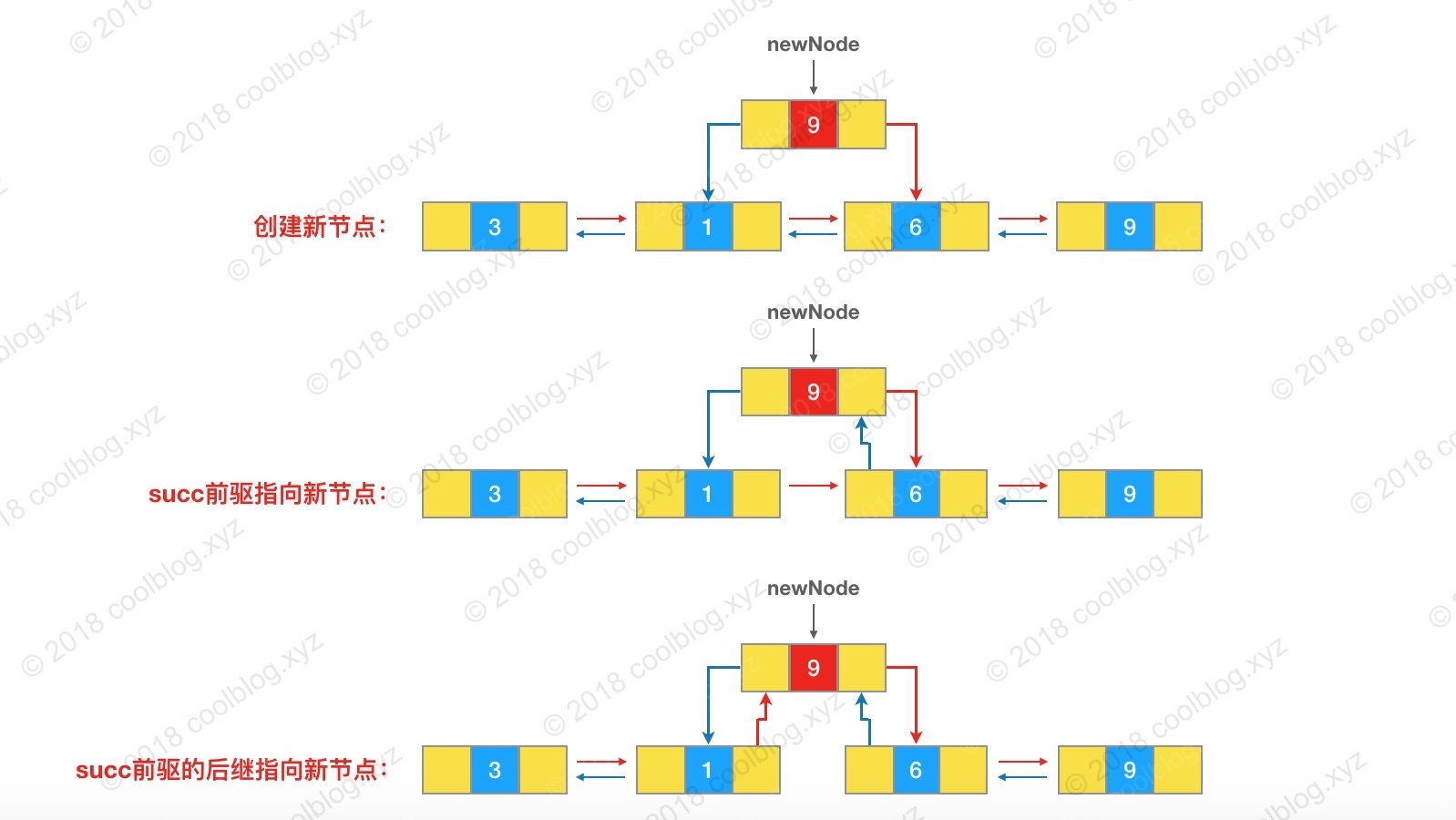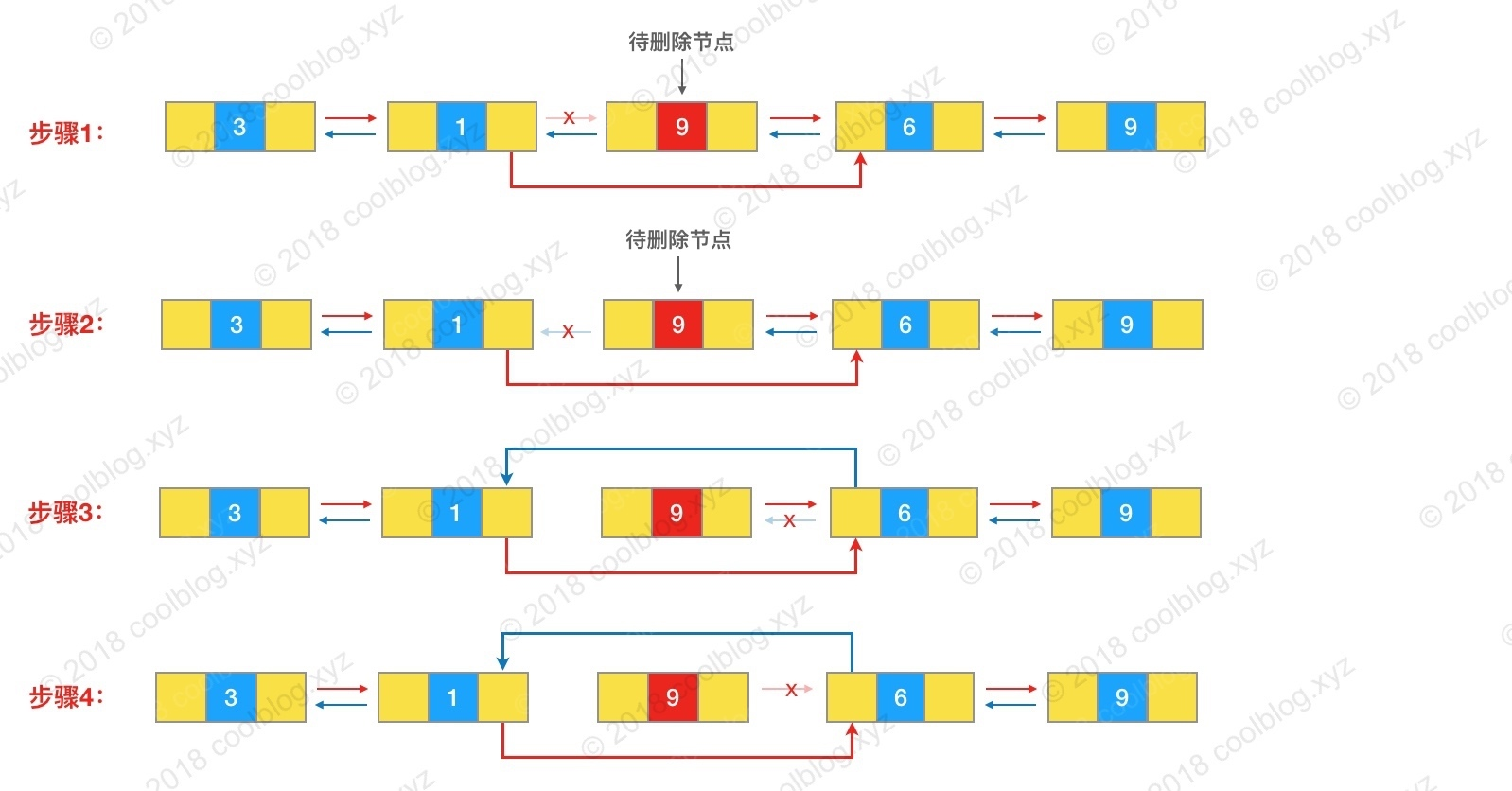Posted by 陈树义 on 2019-08-26

``````public class LinkedList<E>
extends AbstractSequentialList<E>
implements List<E>, Deque<E>, Cloneable, java.io.Serializable
``````

## 原理

### 类成员变量

``````// 链表大小
transient int size = 0;
// 首节点
transient Node<E> first;
// 尾节点
transient Node<E> last;
// Node节点
private static class Node<E> {
E item;
Node<E> next;
Node<E> prev;

Node(Node<E> prev, E element, Node<E> next) {
this.item = element;
this.next = next;
this.prev = prev;
}
}
``````

### 构造方法

``````public LinkedList() {
}
public LinkedList(Collection<? extends E> c) {
this();
}
``````

### 核心方法

#### 查找

``````public E get(int index) {
checkElementIndex(index);
return node(index).item;
}

Node<E> node(int index) {
/*
* 如果获取的元素小于容量的一般，则从头结点开始查找，否则从尾节点开始查找。
*/
if (index < (size >> 1)) {
Node<E> x = first;
// 循环向后查找，直至 i == index
for (int i = 0; i < index; i++)
x = x.next;
return x;
} else {
Node<E> x = last;
for (int i = size - 1; i > index; i--)
x = x.prev;
return x;
}
}
``````

#### 插入

``````public boolean add(E e) {
return true;
}
``````

``````void linkLast(E e) {
final Node<E> l = last;
final Node<E> newNode = new Node<>(l, e, null);
last = newNode;
if (l == null)
first = newNode;
else
l.next = newNode;
size++;
modCount++;
}
``````

``````public void add(int index, E element) {
checkPositionIndex(index);

if (index == size)
else
}
``````

``````void linkBefore(E e, Node<E> succ) {
// assert succ != null;
final Node<E> pred = succ.prev;
final Node<E> newNode = new Node<>(pred, e, succ);
succ.prev = newNode;
if (pred == null)
first = newNode;
else
pred.next = newNode;
size++;
modCount++;
}
``````#### 删除

``````public boolean remove(Object o) {
if (o == null) {
for (Node<E> x = first; x != null; x = x.next) {
if (x.item == null) {
return true;
}
}
} else {
for (Node<E> x = first; x != null; x = x.next) {
if (o.equals(x.item)) {
return true;
}
}
}
return false;
}
``````

``````E unlink(Node<E> x) {
// assert x != null;
final E element = x.item;
final Node<E> next = x.next;
final Node<E> prev = x.prev;

if (prev == null) {
first = next;
} else {
prev.next = next;
x.prev = null;
}

if (next == null) {
last = prev;
} else {
next.prev = prev;
x.next = null;
}

x.item = null;
size--;
modCount++;
return element;
}
``````## 总结

• 底层基于链表实现，修改速度快，读取速度慢（读取时间复杂度O(N)，修改时间复杂度O(N)，因为要查找元素，所以修改也是O(N)）。
• 非线程安全。